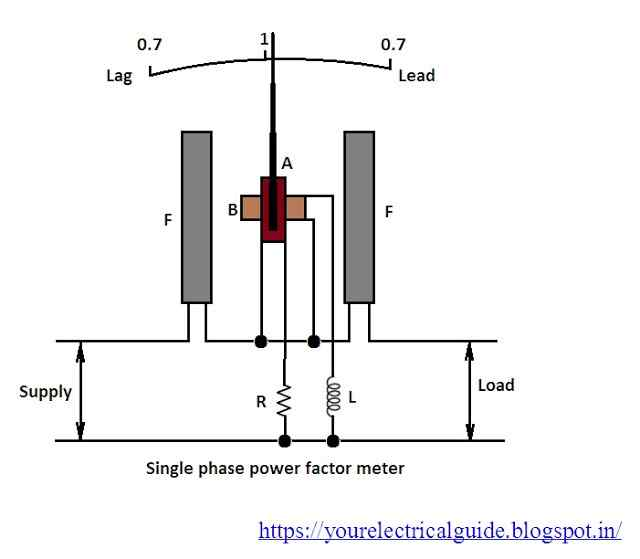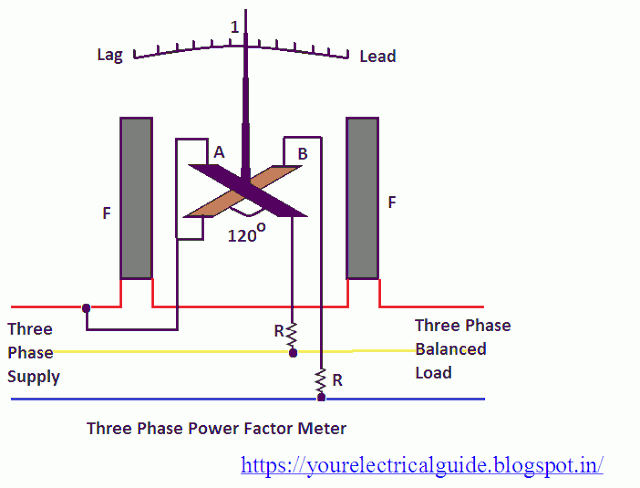# Power Factor Meter

Thepower factor of a circuit can be found out from the wattmeter reading and voltmeter and ammeter readings suitably connected in the circuit.

Power factor = Wattmeter reading / (Voltmeter reading x ammeter reading).

Power factor = True Power / Apparent Power

This method involves mathematical calculations. Sometimes it is required to measure the power factor of the circuit instantaneously when the power factor of the load is varying continuously.

This purpose is served by connecting a dynamometer type power factor meter in the circuit. It indicates the power factor of the circuit directly on the scale by the deflection of a pointer.

# Power Factor Meter Working Principle

The basic working principle of power factor meter is similar to that of dynamometer type wattmeter i.e. when the field produced by moving system tries to come in line with the field produced by the fixed coil, deflecting torque is exerted on the moving system which deflects the pointer attached to it (the moving system).

## Construction of a Power Factor Meter

A dynamometer type power factor meter consists of two fixed coils FF connected in series carrying the load current (or a definite fraction of it) forming the current circuit, and two identical moving coils A and B fixed at the nearly right angle to each other pivoted on the same spindle forming the pressure circuit as shown in the figure.The current coils FF are wound with thick wire whereas pressure coils A and B are wound with fine wire. The pressure coils fixed on the same spindle, to which a pointer is attached, constitutes the moving system.

The pressure coil A is connected across the supply through a non-inductive resistant and pressure coil B is connected across the supply through highly inductive choke coil of inductance L. The value of resistance R and inductance L are so chosen that for the main supply frequency, the current in the two pressure coils A and B is the same.

Thus the fields produced by the two coils are of the same strength. The field produced by the coil B lags behind the field produced by the coil A slightly less than 90° because of resistance of the coil. Accordingly while fixing the coil B the plane of this coil is displaced from the plane of the coil A by the electrical angle which is slightly less than 90°.

However while discussing the action (working) of the instrument it will be assumed that the phase difference between the two currents flowing through the coils A and B is 90° and same is the angle between the planes of the coils.

Though power factor meter is an indicating instrument but no controlling torque is provided in this instrument. The currents are being led into the moving coils A and B by fine ligaments which exert no control.

## Power Factor Meter Working

Since no controlling torque is provided in this instrument, therefore, when it is not connected in the circuit, the moving coils will remain in the position in which these are turned. This will only happen when the moving system is perfectly balanced.

When the instrument is connected to the load circuit, current flows through the fixed coils FF and Moving coils A and B, flux is set by the fixed coils and moving coils.

By the alignment of two fields, torque develops i.e. the resultant field produced by the moving coils tries to come in line with the field produced by the fixed coils and torque develops till both of them come in line with each other. There are three extreme conditions in which this instrument is connected in the circuit.

1. When power factor of the circuit is unity: In this case, current is in phase with circuit voltage. The current flowing through potential coil A is in phase with the voltage which is also in phase with the current flowing through current coil FF.

At the same time, the current flowing through potential coil B lags behind voltage as well as the current flowing through current coil FF by 90o. Thus pressure coil A will experience a turning moment so its plane will come in position a parallel to the plane of the current coil FF. The torque acting on the pressure coil B is zero. Thus, the pointer indicates unity power factor on the scale.
2.

3. When power factor of the circuit is zero lagging: In this case, current lags behind the circuit voltage by 90°. Therefore, the current flowing through pressure coil B will be in phase with the current in current coils FF, both being lagging behind the circuit voltage by 90°.

The current flowing through pressure coil A will lead the current in current coil FF by 90°. Thus a turning moment acts on the pressure coil B and brings its plane parallel to the plane of current coil FF and pointer indicates zero power factor lagging.
4.

5. When power factor of the circuit is zero leading: In this case current leads the circuit voltage by 90o. Therefore, the current flowing through pressure coil A lags the current in current coil FF by 90° and the current flowing through pressure coil B lags the current in current coil FF by 180°.

Thus field produced by the moving system is just reversed to that in the case (2). Thus an opposite turning moment acts on the pressure coil B and brings its plane parallel to the plane of current coil FF and pointer indicates zero power factor leading.

For intermediate power factors the moving system of power factor meter takes up intermediate positions and the pointer indicates the power factor accordingly.

## 3 Phase Power Factor Meter

A dynamometer type three-phase power factor meter gives correct readings only when the load is balanced. The basic principle of this instrument is the same as that of the single-phase dynamometer type power factor meter. The only difference is in its construction.

## Construction of a 3 Phase Power Factor Meter

It consists of two fixed coils FF connected in series in one of the phases and carries the line current as shown in the figure.The two identical moving coils A and B are fixed with their planes 120° apart and are connected across the two remaining phases respectively through high resistances as shown in the figure.

In this case, there is no necessity for phase splitting by artificial means, since the required phase displacement between the currents in the moving coils can be obtained from the supply itself.

## 3 Phase Power Factor Meter Working Principle

When the three phase power factor meter is connected in the circuit, under balanced load conditions, the angle through which the pointer is deflected from the unity power factor position is equal to the phase angle of the circuit, because the two moving coils are fixed 120° apart.

The deflections in three phase power factor meters are independent of frequency and waveform since the currents in the two moving coils are affected in the same way by any change of frequency.

## Power Factor Meter Connection Diagram

Power factor meter connection is shown in Figure.Thanks for reading about power factor meter working principle. Please share, if you liked my work.

## Electrical Measuring Instruments | All Posts

© //www.yourelectricalguide.com/ dynamometer type power factor meter working principle.

### 5 thoughts on “Power Factor Meter”

1.Saya Negi

Helo sir i need to ask you something vould you please help me by clearing my doubts.. i shall be very thankful if you will help me

2.Ajay Sharma

1.Saya Negi

Sir I want to know the torque equation of power factor meter ( electrodynamics type ) and moving iron type

3.Saya Negi

Could you please help me by giving me sufficient information of this subject

1.Ajay Sharma

I have emailed an e book for you on this subject on sayanegi****@gmail.com. Download it and study carefully.